# Polyomino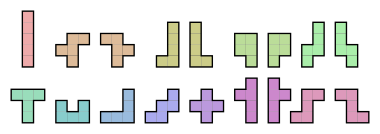The 18 one-sided pentominoes, including 6 mirrored pairs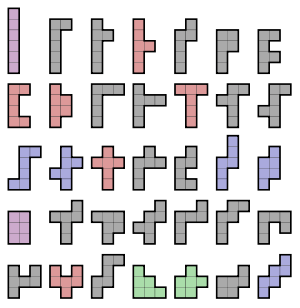The 35 free hexominoes, colored according to their symmetry.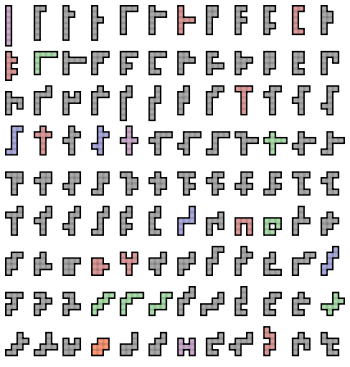The 108 free heptominoes.The single free domino.

A polyomino is a plane geometric figure formed by joining one or more equal squares edge to edge. It is a polyform whose cells are squares. It may be regarded as a finite subset of the regular square tiling with a connected interior.

Polyominoes are classified according to how many cells they have:

Number of cells Name
1 monomino
2 domino
3 tromino
4 tetromino
5 pentomino
6 hexomino
7 heptomino
8 octomino
9 nonomino
10 decomino
11 undecomino
12 dodecomino

Polyominoes have been used in popular puzzles since at least 1907, and the enumeration of pentominoes is dated to antiquity. Many results with the pieces of 1 to 6 squares were first published in Fairy Chess Review between the years 1937 to 1957, under the name of "dissection problems." The name polyomino was invented by Solomon W. Golomb in 1953 and it was popularized by Martin Gardner in his November 1960 "Mathematical Games column" in Scientific American.

Related to polyominoes are polyiamonds, formed from equilateral triangles; polyhexes, formed from regular hexagons; and other plane polyforms. Polyominoes have been generalized to higher dimensions by joining cubes to form polycubes, or hypercubes to form polyhypercubes.

Like many puzzles in recreational mathematics, polyominoes raise many combinatorial problems. The most basic is enumerating polyominoes of a given size. No formula has been found except for special classes of polyominoes. A number of estimates are known, and there are algorithms for calculating them.

Polyominoes with holes are inconvenient for some purposes, such as tiling problems. In some contexts polyominoes with holes are excluded, allowing only simply connected polyominoes.

## Enumeration of polyominoes

### Free, one-sided, and fixed polyominoes

There are three common ways of distinguishing polyominoes for enumeration:

• free polyominoes are distinct when none is a rigid transformation (translation, rotation, reflection or glide reflection) of another (pieces that can be picked up and flipped over). Translating, rotating, reflecting, or glide reflecting a free polyomino does not change its shape.
• one-sided polyominoes are distinct when none is a translation or rotation of another (pieces that cannot be flipped over). Translating or rotating a one-sided polyomino does not change its shape.
• fixed polyominoes are distinct when none is a translation of another (pieces that can be neither flipped nor rotated). Translating a fixed polyomino will not change its shape.

The following table shows the numbers of polyominoes of various types with n cells.

n name (OEIS sequence) free (A000105) free with holes (A001419) free without holes (A000104) one-sided (A000988) fixed (A001168)
1 monomino 1 0 1 1 1
2 domino 1 0 1 1 2
3 tromino 2 0 2 2 6
4 tetromino 5 0 5 7 19
5 pentomino 12 0 12 18 63
6 hexomino 35 0 35 60 216
7 heptomino 108 1 107 196 760
8 octomino 369 6 363 704 2,725
9 nonomino 1,285 37 1,248 2,500 9,910
10 decomino 4,655 195 4,460 9,189 36,446
11 undecomino 17,073 979 16,094 33,896 135,268
12 dodecomino 63,600 4,663 58,937 126,759 505,861

As of 2004, Iwan Jensen has enumerated the fixed polyominoes up to n = 56; the number of fixed polyominoes with 56 cells is approximately 6.915×1031. Free polyominoes have been enumerated up to n = 28 by Tomás Oliveira e Silva.

### Symmetries of polyominoes

The dihedral group D4 is the group of symmetries (symmetry group) of a square. This group contains four rotations and four reflections. It is generated by alternating reflections about the x-axis and about a diagonal. One free polyomino corresponds to at most 8 fixed polyominoes, which are its images under the symmetries of D4. However, those images are not necessarily distinct: the more symmetry a free polyomino has, the fewer distinct fixed counterparts it has. Therefore, a free polyomino that is invariant under some or all non-trivial symmetries of D4 may correspond to only 4, 2 or 1 fixed polyominoes. Mathematically, free polyominoes are equivalence classes of fixed polyominoes under the group D4.

Polyominoes have the following possible symmetries; the least number of squares needed in a polyomino with that symmetry is given in each case:

• 8 fixed polyominoes for each free polyomino:
• no symmetry (4)
• 4 fixed polyominoes for each free polyomino:
• mirror symmetry with respect to one of the grid line directions (4)
• mirror symmetry with respect to a diagonal line (3)
• 2-fold rotational symmetry: C2 (4)
• 2 fixed polyominoes for each free polyomino:
• symmetry with respect to both grid line directions, and hence also 2-fold rotational symmetry: D2 (2)
• symmetry with respect to both diagonal directions, and hence also 2-fold rotational symmetry: D2 (7)
• 4-fold rotational symmetry: C4 (8)
• 1 fixed polyomino for each free polyomino:
• all symmetry of the square: D4 (1).

The following table shows the numbers of polyominoes with n squares, sorted by symmetry groups.

n none (A006749) mirror (90°) (A006746) mirror (45°) (A006748) C2 (A006747) D2 (90°) (A056877) D2 (45°) (A056878) C4 (A144553) D4 (A142886)
1 0 0 0 0 0 0 0 1
2 0 0 0 0 1 0 0 0
3 0 0 1 0 1 0 0 0
4 1 1 0 1 1 0 0 1
5 5 2 2 1 1 0 0 1
6 20 6 2 5 2 0 0 0
7 84 9 7 4 3 1 0 0
8 316 23 5 18 4 1 1 1
9 1,196 38 26 19 4 0 0 2
10 4,461 90 22 73 8 1 0 0
11 16,750 147 91 73 10 2 0 0
12 62,878 341 79 278 15 3 3 3

### Algorithms for enumeration of fixed polyominoes

#### Inductive algorithms

Each polyomino of order n+1 can be obtained by adding a square to a polyomino of order n. This leads to algorithms for generating polyominoes inductively.

Most simply, given a list of polyominoes of order n, squares may be added next to each polyomino in each possible position, and the resulting polyomino of order n+1 added to the list if not a duplicate of one already found; refinements in ordering the enumeration and marking adjacent squares that should not be considered reduce the number of cases that need to be checked for duplicates. This method may be used to enumerate either free or fixed polyominoes.

A more sophisticated method, described by Redelmeier, has been used by many authors as a way of not only counting polyominoes (without requiring that all polyominoes of order n be stored in order to enumerate those of order n+1), but also proving upper bounds on their number. The basic idea is that we begin with a single square, and from there, recursively add squares. Depending on the details, it may count each n-omino n times, once from starting from each of its n squares, or may be arranged to count each once only.

The simplest implementation involves adding one square at a time. Beginning with an initial square, number the adjacent squares, clockwise from the top, 1, 2, 3, and 4. Now pick a number between 1 and 4, and add a square at that location. Number the unnumbered adjacent squares, starting with 5. Then, pick a number larger than the previously picked number, and add that square. Continue picking a number larger than the number of the current square, adding that square, and then numbering the new adjacent squares. When n squares have been created, an n-omino has been created.

This method ensures that each fixed polyomino is counted exactly n times, once for each starting square. It can be optimized so that it counts each polyomino only once, rather than n times. Starting with the initial square, declare it to be the lower-left square of the polyomino. Simply do not number any square that is on a lower row, or left of the square on the same row. This is the version described by Redelmeier.

If one wishes to count free polyominoes instead, then one may check for symmetries after creating each n-omino. However, it is faster to generate symmetric polyominoes separately (by a variation of this method) and so determine the number of free polyominoes by Burnside's lemma.

#### Transfer-matrix method

The most modern algorithm for enumerating the fixed polyominoes was discovered by Iwan Jensen. An improvement on Andrew Conway's method, it is exponentially faster than the previous methods (however, its running time is still exponential in n).

Both Conway's and Jensen's versions of the transfer-matrix method involve counting the number of polyominoes that have a certain width. Computing the number for all widths gives the total number of polyominoes. The basic idea behind the method is that possible beginning rows are considered, and then to determine the minimum number of squares needed to complete the polyomino of the given width. Combined with the use of generating functions, this technique is able to count many polyominoes at once, thus enabling it to run many times faster than methods that have to generate every polyomino.

Although it has excellent running time, the tradeoff is that this algorithm uses exponential amounts of memory (many gigabytes of memory are needed for n above 50), is much harder to program than the other methods, and can't currently be used to count free polyominoes.

### Asymptotic growth of the number of polyominoes

#### Fixed polyominoes

Theoretical arguments and numerical calculations support the estimate for the number of fixed polyominoes of size nwhere λ = 4.0626 and c = 0.3169. However, this result is not proven and the values of λ and c are only estimates.

The known theoretical results are not nearly as specific as this estimate. It has been proven thatexists. In other words, An grows exponentially. The best known lower bound for λ is 3.980137. The best known upper bound, not improved since the 1970s, is λ < 4.65.

To establish a lower bound, a simple but highly effective method is concatenation of polyominoes. Define the upper-right square to be the rightmost square in the uppermost row of the polyomino. Define the bottom-left square similarly. Then, the upper-right square of any polyomino of size n can be attached to the bottom-left square of any polyomino of size m to produce a unique (n+m)-omino. This proves AnAm An+m. Using this equation, one can show λ (An)1/n for all n. Refinements of this procedure combined with data for An produce the lower bound given above.

The upper bound is attained by generalizing the inductive method of enumerating polyominoes. Instead of adding one square at a time, one adds a cluster of squares at a time. This is often described as adding twigs. By proving that every n-omino is a sequence of twigs, and by proving limits on the combinations of possible twigs, one obtains an upper bound on the number of n-ominoes. For example, in the algorithm outlined above, at each step we must choose a larger number, and at most three new numbers are added (since at most three unnumbered squares are adjacent to any numbered square). This can be used to obtain an upper bound of 6.75. Using 2.8 million twigs, Klarner and Rivest obtained an upper bound of 4.65.

#### Free polyominoes

Approximations for the number of fixed polyominoes and free polyominoes are related in a simple way. A free polyomino with no symmetries (rotation or reflection) corresponds to 8 distinct fixed polyominoes, and for large n, most n-ominoes have no symmetries. Therefore, the number of fixed n-ominoes is approximately 8 times the number of free n-ominoes. Moreover, this approximation is exponentially more accurate as n increases.

### Special classes of polyominoes

Exact formulas are known for enumerating polyominoes of special classes, such as the class of convex polyominoes and the class of directed polyominoes.

The definition of a convex polyomino is different from the usual definition of convexity, but is similar to the definition used for the orthogonal convex hull. A polyomino is said to be vertically or column convex if its intersection with any vertical line is convex (in other words, each column has no holes). Similarly, a polyomino is said to be horizontally or row convex if its intersection with any horizontal line is convex. A polyomino is said to be convex if it is row and column convex.

A polyomino is said to be directed if it contains a square, known as the root, such that every other square can be reached by movements of up or right one square, without leaving the polyomino.

Directed polyominoes, column (or row) convex polyominoes, and convex polyominoes have been effectively enumerated by area n, as well as by some other parameters such as perimeter, using generating functions.

A polyomino is equable if its area equals its perimeter. An equable polyomino must be made from an even number of squares; every even number greater than 15 is possible. For instance, the 16-omino in the form of a 4 × 4 square and the 18-omino in the form of a 3 × 6 rectangle are both equable. For polyominoes with fewer than 15 squares, the perimeter always exceeds the area.

## Uses of polyominoes

Polyominoes have fostered research in computer science and recreational mathematics. Challenges are often posed for covering (tiling) a prescribed region, or the entire plane, with polyominoes, or folding a polyomino to create other shapes. Gardner proposed several simple games with a set of free pentominoes and a chessboard. Some variants of the Sudoku puzzle use polyomino-shaped regions on the grid. The video game Tetris is based on the seven one-sided tetrominoes (spelled "Tetriminos" in the game), and the board game Blokus uses all of the free polyominoes up to pentominoes.

### Tiling regions with sets of polyominoes

Puzzles commonly ask for tiling a given region with a given set of polyominoes, such as the 12 pentominoes. Golomb's and Gardner's books have many examples. A typical puzzle is to tile a 6×10 rectangle with the twelve pentominoes; the 2339 solutions to this were found in 1960. Where multiple copies of the polyominoes in the set are allowed, Golomb defines a hierarchy of different regions that a set may be able to tile, such as rectangles, strips, and the whole plane, and shows that whether polyominoes from a given set can tile the plane is undecidable, by mapping sets of Wang tiles to sets of polyominoes.

### Tiling regions with copies of a single polyomino

Another class of problems asks whether copies of a given polyomino can tile a rectangle, and if so, what rectangles they can tile. These problems have been extensively studied for particular polyominoes, and tables of results for individual polyominoes are available. Klarner and Göbel showed that for any polyomino there is a finite set of prime rectangles it tiles, such that all other rectangles it tiles can be tiled by those prime rectangles.

Beyond rectangles, Golomb gave his hierarchy for single polyominoes: a polyomino may tile a rectangle, a half strip, a bent strip, an enlarged copy of itself, a quadrant, a strip, a half plane, the whole plane, certain combinations, or none of these. There are certain implications among these, both obvious (for example, if a polyomino tiles the half plane then it tiles the whole plane) and less so (for example, if a polyomino tiles an enlarged copy of itself, then it tiles the quadrant). Polyominoes of orders up to 6 are characterized in this hierarchy (with the status of one hexomino, later found to tile a rectangle, unresolved at that time).

In 2001 Cristopher Moore and John Michael Robson showed that the problem of tiling one polyomino with copies of another is NP-complete.

### Tiling the plane with copies of a single polyomino

Tiling the plane with copies of a single polyomino has also been much discussed. It was noted in 1965 that all polyominoes up to hexominoes and all but four heptominoes tile the plane. It was then established by David Bird that all but 26 octominoes tile the plane. Rawsthorne found that all but 235 polyominoes of order 9 tile, and such results have been extended to higher orders by Rhoads (to order 14) and others. Polyominoes tiling the plane have been classified by the symmetries of their tilings and by the number of aspects (orientations) in which the tiles appear in them.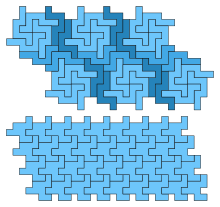The two tiling nonominoes not satisfying the Conway criterion.

The study of which polyominoes can tile the plane has been facilitated using the Conway criterion: except for two nonominoes, all tiling polyominoes up to order 9 form a patch of at least one tile satisfying it, with higher-order exceptions more frequent.

Several polyominoes can tile larger copies of themselves, and repeating this process recursively gives a rep-tile tiling of the plane. For instance, for every positive integer n, it is possible to combine n2 copies of the L-tromino, L-tetromino, or P-pentomino into a single larger shape similar to the smaller polyomino from which it was formed.

### Tiling a common figure with various polyominoes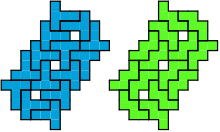A minimal compatibility figure for the T and W pentominoes.

The compatibility problem is to take two or more polyominoes and find a figure that can be tiled with each. Polyomino compatibility has been widely studied since the 1990s. Jorge Luis Mireles and Giovanni Resta have published websites of systematic results, and Livio Zucca shows results for some complicated cases like three different pentominoes. The general problem can be hard. The first compatibility figure for the L and X pentominoes was published in 2005 and had 80 tiles of each kind. Many pairs of polyominoes have been proved incompatible by systematic exhaustion. No algorithm is known for deciding whether two arbitrary polyominoes are compatible.

## Etymology

The word polyomino and the names of the various orders of polyomino are all back-formations from the word domino, a common game piece consisting of two squares, with the first letter d- fancifully interpreted as a version of the prefix di- meaning "two." The name domino for the game piece is believed to come from the spotted masquerade garment domino, from Latin dominus.

Most of the numerical prefixes are Greek. Polyominoes of order 9 and 11 more often take the Latin prefixes nona- (nonomino) and undeca- (undecomino) than the Greek prefixes ennea- (enneomino) and hendeca- (hendecomino).

• Percolation theory, the mathematical study of random subsets of integer grids. The finite connected components of these subsets form polyominoes.
• Young diagram, a special kind of polyomino used in number theory to describe integer partitions and in group theory and applications in mathematical physics to describe representations of the symmetric group.
• Blokus, a board game using polyominoes.
• Squaregraph, a kind of undirected graph including as a special case the graphs of vertices and edges of polyominoes.

## Notes

1. Golomb (Polyominoes, Preface to the First Edition) writes "the observation that there are twelve distinctive patterns (the pentominoes) that can be formed by five connected stones on a Go board is attributed to an ancient master of that game".
2. Golomb, Solomon W. (1994). Polyominoes (2nd ed.). Princeton, New Jersey: Princeton University Press. ISBN 0-691-02444-8.
3. Grünbaum, Branko; Shephard, G.C. (1987). Tilings and Patterns. New York: W.H. Freeman and Company. ISBN 0-7167-1193-1.
4. Redelmeier, D. Hugh (1981). "Counting polyominoes: yet another attack". Discrete Mathematics. 36 (2): 191–203. doi:10.1016/0012-365X(81)90237-5.
5. Golomb, chapter 6
6. Iwan Jensen. "Series for lattice animals or polyominoes". Retrieved 2007-05-06.
7. Tomás Oliveira e Silva. "Animal enumerations on the {4,4} Euclidean tiling". Retrieved 2007-05-06.
8. Redelmeier, section 3
9. Golomb, pp. 73–79
10. Redelmeier, section 4
11. Redelmeier, section 6
12. Jensen, Iwan (February 2001). "Enumerations of Lattice Animals and Trees". Journal of Statistical Physics. 102 (3–4): 865–881. arXiv:cond-mat/0007239. doi:10.1023/A:1004855020556.
13. Conway, Andrew (1995). "Enumerating 2D percolation series by the finite-lattice method: theory". Journal of Physics A: Mathematical and General. 28 (2): 335–349. doi:10.1088/0305-4470/28/2/011. Zbl 0849.05003.
14. Jensen, Iwan; Guttmann, Anthony J. (2000). "Statistics of lattice animals (polyominoes) and polygons". Journal of Physics A: Mathematical and General. 33 (29): L257–L263. doi:10.1088/0305-4470/33/29/102.
15. Barequet, Gill; Moffie, Micha; Ribó, Ares; Rote, Günter (2006). "Counting polyominoes on twisted cylinders". Integers. 6: A22.
16. Klarner, D.A.; Rivest, R.L. (1973). "A procedure for improving the upper bound for the number of n-ominoes" (PDF of technical report version). Canadian Journal of Mathematics. 25: 585–602. doi:10.4153/CJM-1973-060-4. Retrieved 2007-05-11.
17. Wilf, Herbert S. (1994). Generatingfunctionology (2nd ed.). Boston, MA: Academic Press. p. 151. ISBN 0-12-751956-4. Zbl 0831.05001.
18. Bousquet-Mélou, Mireille (1998). "New enumerative results on two-dimensional directed animals". Discrete Mathematics. 180 (1–3): 73–106. doi:10.1016/S0012-365X(97)00109-X.
19. Delest, M.-P. (1988). "Generating functions for column-convex polyominoes". Journal of Combinatorial Theory. Series A. 48 (1): 12–31. doi:10.1016/0097-3165(88)90071-4.
20. Bousquet-Mélou, Mireille; Fédou, Jean-Marc (1995). "The generating function of convex polyominoes: The resolution of a q-differential system". Discrete Mathematics. 137 (1–3): 53–75. doi:10.1016/0012-365X(93)E0161-V.
21. Picciotto, Henri (1999), Geometry Labs, MathEducationPage.org, p. 208.
22. Martin, George E. (1996). Polyominoes: A guide to puzzles and problems in tiling (2nd ed.). Mathematical Association of America. ISBN 0-88385-501-1.
23. C.B. Haselgrove; Jenifer Haselgrove (October 1960). "A Computer Program for Pentominoes". Eureka. 23: 16–18.
24. Golomb, Solomon W. (1970). "Tiling with Sets of Polyominoes". Journal of Combinatorial Theory. 9: 60–71. doi:10.1016/S0021-9800(70)80055-2.
25. Golomb, Polyominoes, chapter 8
26. Reid, Michael. "References for Rectifiable Polyominoes". Retrieved 2007-05-11.
27. Reid, Michael. "List of known prime rectangles for various polyominoes". Retrieved 2007-05-11.
28. Klarner, D.A.; Göbel, F. (1969). "Packing boxes with congruent figures". Indagationes Mathematicae. 31: 465–472.
29. Klarner, David A. (February 1973). "A Finite Basis Theorem Revisited" (PDF). Stanford University Technical Report STAN-CS-73–338. Retrieved 2007-05-12.
30. Golomb, Solomon W. (1966). "Tiling with Polyominoes". Journal of Combinatorial Theory. 1 (2): 280–296. doi:10.1016/S0021-9800(66)80033-9.
31. Moore, Cristopher; Robson, John Michael (2001). "Hard Tiling Problems with Simple Tiles" (PDF).
32. Petersen, Ivars (September 25, 1999), "Math Trek: Tiling with Polyominoes", Science News.
33. Gardner, Martin (July 1965). "On the relation between mathematics and the ordered patterns of Op art". Scientific American. 213 (1): 100–104.
34. Gardner, Martin (August 1965). "Thoughts on the task of communication with intelligent organisms on other worlds". Scientific American. 213 (2): 96–100.
35. Gardner, Martin (August 1975). "More about tiling the plane: the possibilities of polyominoes, polyiamonds and polyhexes". Scientific American. 233 (2): 112–115.
36. Rawsthorne, Daniel A. (1988). "Tiling complexity of small n-ominoes (n<10)". Discrete Mathematics. 70: 71–75. doi:10.1016/0012-365X(88)90081-7.
37. Rhoads, Glenn C. (2003). Planar Tilings and the Search for an Aperiodic Prototile. PhD dissertation, Rutgers University.
38. Grünbaum and Shephard, section 9.4
39. Keating, K.; Vince, A. (1999). "Isohedral Polyomino Tiling of the Plane". Discrete & Computational Geometry. 21 (4): 615–630. doi:10.1007/PL00009442.
40. Rhoads, Glenn C. (2005). "Planar tilings by polyominoes, polyhexes, and polyiamonds". Journal of Computational and Applied Mathematics. 174 (2): 329–353. doi:10.1016/j.cam.2004.05.002.
41. Niţică, Viorel (2003). "Rep-tiles revisited". MASS selecta. Providence, RI: American Mathematical Society. pp. 205–217. MR 2027179.
42. Mireles, J.L., "Poly2ominoes"
43. Resta, G., "Polypolyominoes"
44. Zucca, L., "Remembrance of Software Past"
45. Barbans, Uldis; Cibulis, Andris; Lee, Gilbert; Liu, Andy; Wainwright, Robert (2005). "Polyomino Number Theory (III)". In Cipra, Barry; Demaine, Erik D.; Demaine, Martin L.; Rodgers, Tom. Tribute to a Mathemagician. Wellesley, MA: A.K. Peters. pp. 131–136. ISBN 978-1-56881-204-5.
46. Oxford English Dictionary, 2nd edition, entry domino